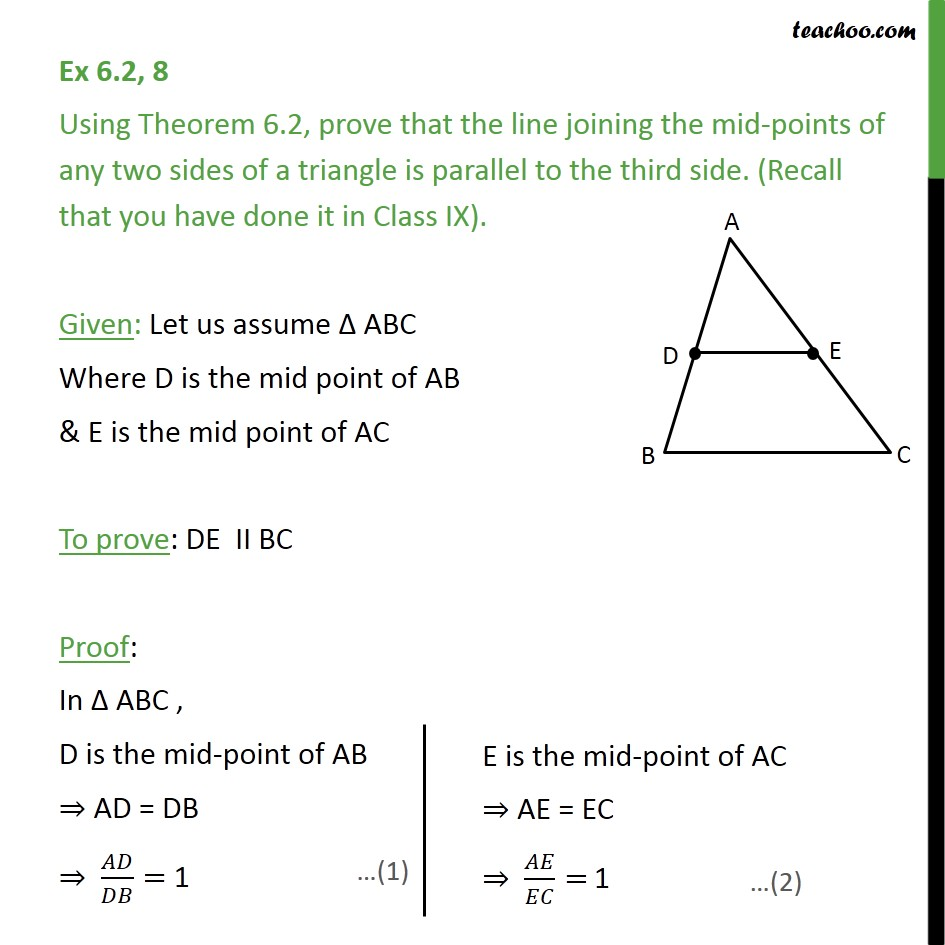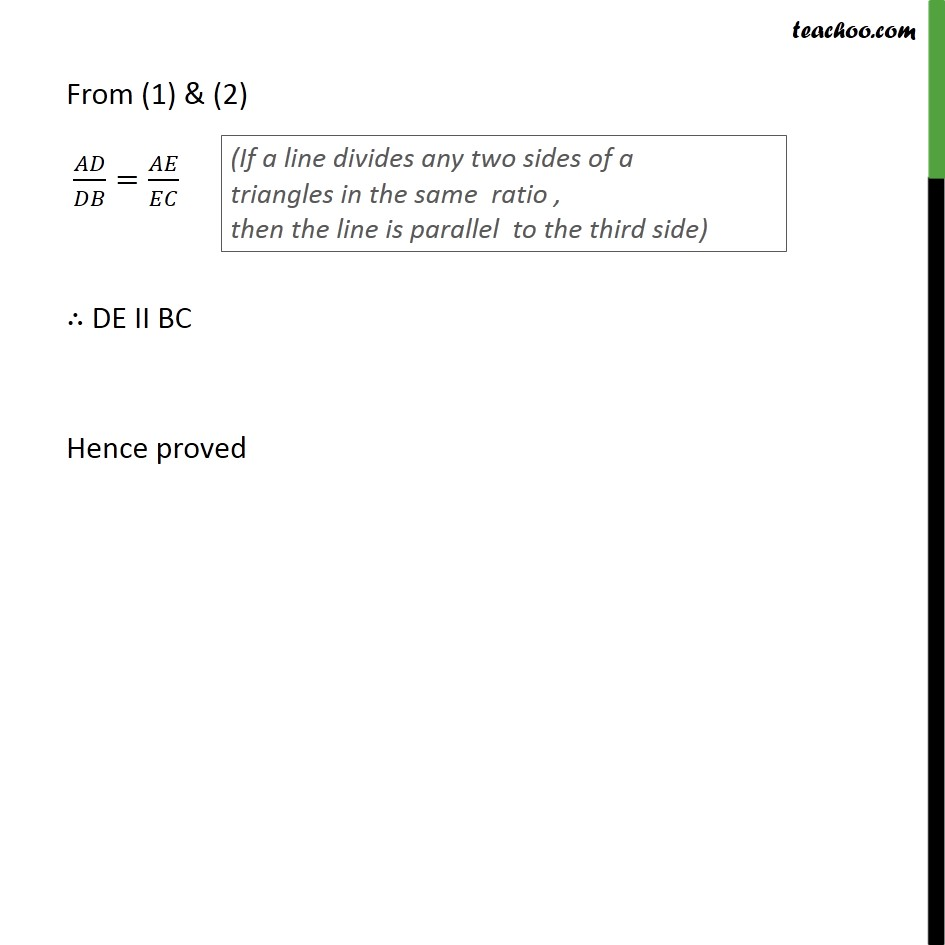Ex 6.2

Chapter 6 Class 10 Triangles
Serial order wiseLearn in your speed, with individual attention - Teachoo Maths 1-on-1 Class

### Transcript

Ex 6.2, 8 Using Theorem 6.2, prove that the line joining the mid-points of any two sides of a triangle is parallel to the third side. (Recall that you have done it in Class IX). Given: Let us assume Δ ABC Where D is the mid point of AB & E is the mid point of AC To prove: DE II BC Proof: In Δ ABC , D is the mid-point of AB ⇒ AD = DB ⇒ 𝐴𝐷/𝐷𝐵= 1 From (1) & (2) 𝐴𝐷/𝐷𝐵=𝐴𝐸/𝐸𝐶 ∴ DE II BC Hence proved